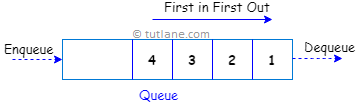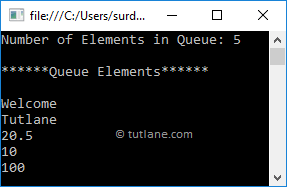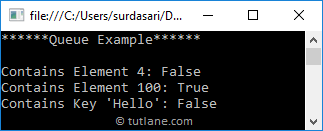# Visual Basic Queue

In visual basic, Queue is useful to represent a collection of objects which stores elements in FIFO (First In, First out) style i.e. the element which added first will come out first. In queue, elements are inserted from one end and removed from another end.

Generally, queues are useful when we want to access elements from the collection in the same order that is stored and we can store a multiple null and duplicate values in the queue based on our requirements.

By using Enqueue() and Dequeue() methods, we can add or delete elements from the queue. Here, the Enqueue() method is useful to add elements at the end of the queue and the Dequeue() method is useful to remove elements start from the queue.

Following is the pictorial representation of the queue process flow in a visual basic programming language.## Visual Basic Queue Declaration

Generally, the visual basic will support both generic and non-generic types of queues. Here, we will learn about non-generic queue collections by using the System.Collections namespace.

As discussed, the collection is a class so to define a queue, we must need to declare an instance of the queue class before we perform any operations like add, delete, etc. like as shown below.

Dim que As Queue = New Queue();

If you observe the above queue declaration, we created a new queue (que) with an instance of the queue class without specifying any size.

## Visual Basic Queue Properties

The following are some of the commonly used properties of a queue in visual basic programming language.

PropertyDescription
Count It will return the total number of elements in the queue
IsSynchronized It is used to get a value to indicate that access to the queue is synchronized (thread-safe) or not.

## Visual Basic Queue Methods

The following are some of the commonly used methods of the queue to perform operations like add, delete, etc. on elements of a queue in c# programming language.

MethodDescription
Enqueue It is used to add elements at the end of the queue.
Dequeue It will remove and returns an item from the starting of queue.
Clear It will remove all the elements from the queue.
Clone It will create a shallow copy of the queue.
Contains It is used determine whether an element exists in queue or not.
Peek It is used to get the first element from the queue.
TrimToSize It is used to set the capacity of the queue to an actual number of elements in the queue.

## Visual Basic Queue Example

Here, we are going to use a non-generic type of queue so we can add elements of different data types to the queue using Enqueue() method. Following is the example of adding and accessing elements of the queue in visual basic.

Module Module1

Sub Main(ByVal args As String())

' Create and initialize a queue

Dim que As Queue = New Queue()

que.Enqueue("Welcome")

que.Enqueue("Tutlane")

que.Enqueue(20.5F)

que.Enqueue(10)

que.Enqueue(100)

Console.WriteLine("******Queue Example******")

Console.WriteLine("Number of Elements in Queue: {0}", que.Count)

Console.WriteLine("******Queue Elements******")

' Access Queue Elements

For Each item In que

Console.WriteLine(item)

Next

End Sub

End Module

If you observe the above example, we created a new queue (que) and added different data type elements to the queue (que) using Enqueue() method and we used a For Each loop to iterate through a queue to get elements from it.

When we execute the above visual basic program, we will get the result like as shown below.If you observe the above result, we got elements from the queue in the same order which we added first.

## Visual Basic Dequeue() Method to Access Queue Elements

As discussed, the queue Dequeue() method will always remove and return a first element of the queue. Following is the example of accessing queue elements using the Dequeue() method in visual basic.

Module Module1

Sub Main(ByVal args As String())

' Create and initialize a queue

Dim que As Queue = New Queue()

que.Enqueue("Welcome")

que.Enqueue("Tutlane")

que.Enqueue(20.5F)

que.Enqueue(10)

que.Enqueue(100)

Console.WriteLine("Number of Elements in Queue: {0}", que.Count)

Console.WriteLine("******Queue Elements******")

' Access Queue Elements

While que.Count > 0

Console.WriteLine(que.Dequeue())

End While

Console.WriteLine("Number of Elements in Queue: {0}", que.Count)

End Sub

End Module

If you observe the above example, we are accessing queue elements by using Dequeue() method and calling a Dequeue method on the empty queue will throw an exception (InvalidOperation) so to avoid that situation we are checking whether the count of queue elements greater than zero or not using while loop.

When we execute the above visual basic program, we will get the result like as shown below.If you observe the above result, every time the Dequeue() method has removed and returned the first element of the queue that’s the reason in the end, we got a count of queue elements as 0.

In case, if you want to remove all the elements of the queue, then you need to use the Clear() method.

## Visual Basic Peek() Method to Access Queue Elements

As discussed, the queue Peek() method will always return the first element of the queue. Following is the example of accessing queue elements using the Peek() method in visual basic.

Module Module1

Sub Main(ByVal args As String())

' Create and initialize a queue

Dim que As Queue = New Queue()

que.Enqueue("Welcome")

que.Enqueue("Tutlane")

que.Enqueue(20.5F)

que.Enqueue(10)

Console.WriteLine("Number of Elements in Queue: {0}", que.Count)

Console.WriteLine("******Queue Elements******")

' Access Queue Elements

Console.WriteLine(que.Peek())

Console.WriteLine(que.Peek())

Console.WriteLine(que.Peek())

Console.WriteLine("Number of Elements in Queue: {0}", que.Count)

End Sub

End Module

If you observe the above example, we are accessing queue elements by using the Peek() method.

When we execute the above visual basic program, we will get the result as shown below.

Number of Elements in Queue: 4

******Queue Elements******

Welcome

Welcome

Welcome

Number of Elements in Queue: 4

If you observe the above result, every time the Peek() method has returned a first element of the queue without removing any element that’s the reason at the end also the queue elements count is same as the starting of the queue.

## Visual Basic Queue Contains() Method

In visual basic, by using queue Contains() method we can check whether an element exists in the queue or not. In case, if element found in the queue, then it will return True otherwise False.

Following is the example of using queue Contains() method to check whether an item exists in the queue or not in visual basic.

Module Module1

Sub Main(ByVal args As String())

' Create and initialize a queue

Dim que As Queue = New Queue()

que.Enqueue("Welcome")

que.Enqueue("Tutlane")

que.Enqueue(20.5F)

que.Enqueue(10)

que.Enqueue(100)

Console.WriteLine("******Queue Example******")

Console.WriteLine("Contains Element 4: {0}", que.Contains(4))

Console.WriteLine("Contains Element 100: {0}", que.Contains(100))

Console.WriteLine("Contains Key 'Hello': {0}", que.Contains("Hello"))

End Sub

End Module

If you observe the above example, we used a Contains() method to check for particular elements exists in the queue (que) or not.

When we execute the above visual basic program, we will get the result as shown below.## Visual Basic Queue Overview

Following are the important points which needs to remember about the queue in visual basic.

• In visual basic, queues are useful to store a collection of objects in FIFO (First In, First out) style i.e. the element which added first will come out first.
• By using Enqueue() method, we can add elements at the end of queue.
• The Dequeue() method will remove and return the oldest (first) element from the queue.
• The queue Peek() method will always return a first element of the queue and it won’t delete any element from the queue.